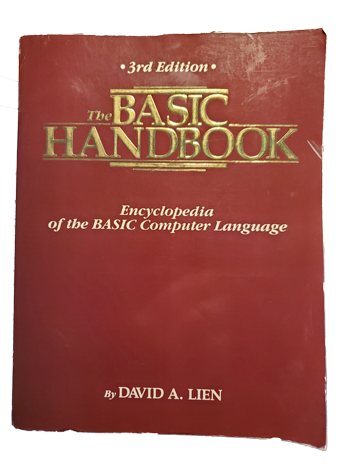# Getting to the Square Root of the Problem

The first part of my writing career began by updating a computer book bestseller, The BASIC Handbook. This tome served as a lexicon for the various BASIC programming language dialects, allowing users from one version of BASIC to translate and use code from another version.Figure 1. The BASIC Handbook, Compusoft Publishing, 1986.

One of the more interesting parts of the book included BASIC code in the form of a generic algorithm. You could use the algorithm in case your version of BASIC lacked a command available for other versions.

For example, if your version of BASIC lacked the absolute value command, `ABS`, you could write your own. From the book:

```29999 END
30000 REM * 'ABS' SUBROUTINE *
30010 REM * INPUT IS X, OUTPUT IS Y *
30020 Y = X
30030 IF X>=0 THEN 30060
30040 Y = -X
30050 RETURN```

Looking back on that code in the book, it’s amazing how sloppy BASIC can be. Further, I found a typo: Line 30030 incorrectly lists Line 30060 as the `RETURN` command. It should be 30050. Ugh.

Generally speaking, C programmers are familiar with this book’s approach for missing functions. C lacks many of the higher-level functions of other languages. So programmers write their own. In fact, sites like https://stackoverflow.com/ are rife with examples of “missing” C language functions.

Still, it’s intriguing for me to read algorithms that handle functions we take for granted. One of these mentioned in the book is the square root command.

From The BASIC Handbook, if your version of BASIC lacked the `SQR` (square root) command you could substitute the following code:

```29999 END
30000 REM * SQUARE ROOT SUBROUTINE *
30010 REM * INPUT X, OUTPUT Y *
30020 REM * ALSO USES W AND Z *
30030 IF X = 0 THEN 30160
30040 IF X>0 THEN 30070
30050 PRINT "ERROR - NEGATIVE ROOT"
30060 STOP
30070 Y = X/4
30080 Z = 0
30090 W = (X/Y-Y)/2
30100 IF W = 0 THEN 30160
30110 IF W = Z THEN 30160
30120 Y = Y + W
30130 Z = W
30140 GOTO 30090
30150 Y = 0
30160 RETURN```

Again, this code has a boner in it: Line 30150 will never be interpreted. I believe the implied `GOTO` at Line 30030 should branch to Line 30150, though it instead goes to the `RETURN` command at Line 30160. Whatever. Because most versions of BASIC implemented the square root (`SQR`) command, no one probably ever used this code. I’m not even certain the testers ran it. I don’t remember.

Anyway, I thought it would be fun to translate this code into C just to see whether it works. After all, code is code; a lot of what’s written above translates directly into C. With a few modifications, I’d roll my own sqrt() function. Wouldn’t that be grand?

As it turns out, the thing didn’t work. I won’t even bother posting my code because the algorithm, at least as I translated it into C, died in an endless loop. The BASIC code above is most likely flawed. Pity.

Not one to give up, I decided to craft my own square root function. I’d start from scratch, determining how a square root is calculated in the first place — well, other than using a calculator, which is probably what I was taught in school.

I explore how to calculate a square root in new week’s Lesson, where I introduce my square root substitute function.

## One thought on “Getting to the Square Root of the Problem”

1. pow(100, 1/2) = 10
pow(1000, 1/3) = 10
etc.